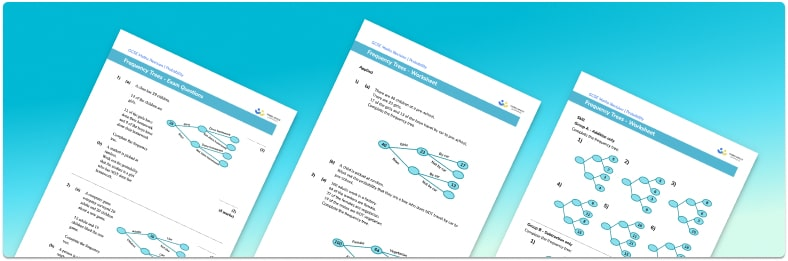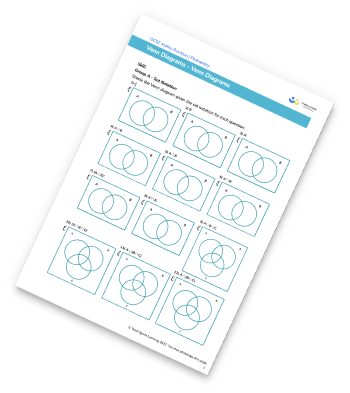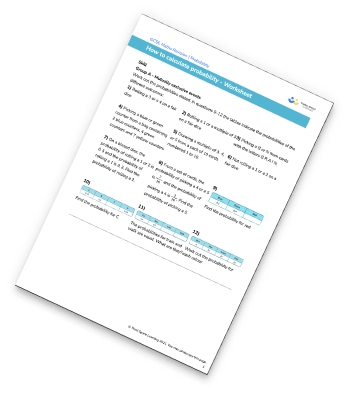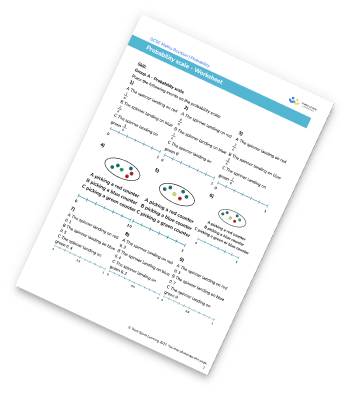# Frequency Trees Worksheet• This field is for validation purposes and should be left unchanged.

You can unsubscribe at any time (each email we send will contain an easy way to unsubscribe). To find out more about how we use your data, see our privacy policy.

• Section 1 of the frequency trees diagram worksheet contains 18 skills-based frequency trees diagram questions, in 3 groups to support differentiation
• Section 2 contains 4 applied frequency trees diagram questions with a mix of worded problems and deeper problem solving questions
• Section 3 contains 3 foundation and higher level GCSE exam style frequency trees diagram questions
• Answers and a mark scheme for all frequency trees diagram questions are provided
• Questions follow variation theory with plenty of opportunities for students to work independently at their own level
• All questions created by fully qualified expert secondary maths teachers
• Suitable for GCSE maths revision for AQA, OCR and Edexcel exam boards

### Frequency trees diagram at a glance

Frequency trees are used to show how a group of objects can be broken down into different categories. Information from two way tables can be written into a frequency tree and vice versa. Frequency trees are useful as they are a form of systematic listing so we can see all of the possible combinations of the categories. Probability questions are common alongside frequency trees as we can calculate different probabilities from a frequency tree.

A frequency tree has a similar structure to a probability tree, however, the frequency of each branch is written at the end of the branch with the total frequency written on the far left of the diagram. The branches are labelled according to their subgroup.

Looking forward, students can then progress to additional probability worksheets, for example a Venn diagram worksheet or

For more teaching and learning support on probability our GCSE maths lessons provide step by step support for all GCSE maths concepts.

## Related worksheets

Venn Diagram WorksheetProbability WorksheetProbability Scale WorksheetVenn Diagram Worksheet## Do you have KS4 students who need more focused attention to succeed at GCSE?There will be students in your class who require individual attention to help them succeed in their maths GCSEs. In a class of 30, it’s not always easy to provide.

Help your students feel confident with exam-style questions and the strategies they’ll need to answer them correctly with our dedicated GCSE maths revision programme.

Lessons are selected to provide support where each student needs it most, and specially-trained GCSE maths tutors adapt the pitch and pace of each lesson. This ensures a personalised revision programme that raises grades and boosts confidence.

Find out more